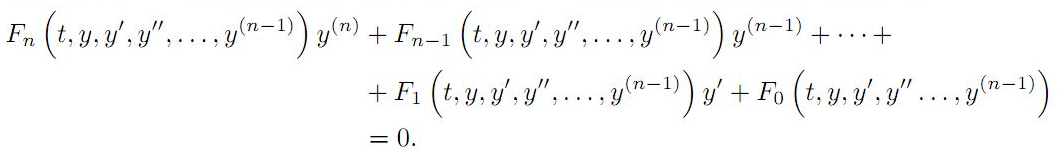The Australian Journal of Mathematical Analysis and Applications

 Home News Editors Volumes RGMIA Subscriptions Authors Contact

ISSN 1449-5910

You searched for almefleh
Total of 4 results found in site

2: Paper Source PDF document

Paper's Title:

Antiderivatives and Integrals Involving Incomplete Beta Functions with Applications

Author(s):

Mathematics Department,
Yarmouk University,
Irbid 21163,
Jordan.
E-mail: rami_thenat@yu.edu.jo

Faculty of Engineering,
Higher Colleges of Technology,
Ras Alkhaimah,
UAE.

Abstract:

In this paper, we prove that incomplete beta functions are antiderivatives of several products and powers of trigonometric functions, we give formulas for antiderivatives for products and powers of trigonometric functions in term of incomplete beta functions, and we evaluate integrals involving trigonometric functions using incomplete beta functions. Also, we extend some properties of the beta functions to the incomplete beta functions. As an application for the above results, we find the moments for certain probability distributions.

1: Paper Source PDF document

Paper's Title:

Integrating Factors and First Integrals of a Class of Third Order Differential Equations

Author(s):

Department of Mathematics,
Yarmouk University,
Irbid 21163,
Jordan.

Abstract:

The principle of finding an integrating factor for a none exact differential equations is extended to a class of third order differential equations. If the third order equation is not exact, under certain conditions, an integrating factor exists which transforms it to an exact one. Hence, it can be reduced into a second order differential equation. In this paper, we give explicit forms for certain integrating factors of a class of the third order differential equations.

1: Paper Source PDF document

Paper's Title:

On Finding Integrating Factors and First Integrals for a Class of Higher Order Differential Equations

Author(s):

Department of Mathematics,
Yarmouk University,
Irbid, 21163,
Jordan.

Abstract:

If the \$n-th\$ order differential equation is not exact, under certain conditions, an integrating factor exists which transforms the differential equation into an exact one. Thus, the order of differential equation can be reduced to the lower order. In this paper, we present a technique for finding integrating factors of the following class of differential equations:Here, the functions F0,F1,F2, ,Fn are assumed to be continuous functions with their first partial derivatives on some simply connected domain Ω Rn+1. We also presented some demonstrative examples

Search and serve lasted 0 second(s).